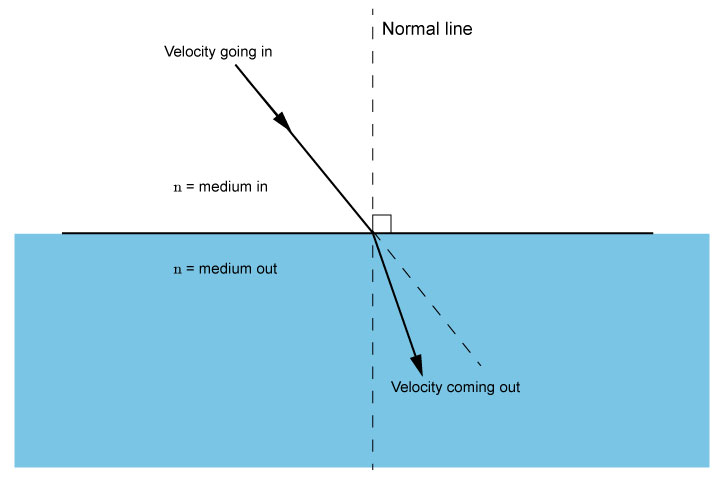# Snell's law applies to velocity

You are unlikely to need this at this level, but it would be useful to know.

Although we know that Snell's law is

(sin\ \i\n\ \theta)/(sin\ \out\ \theta)=(n\ \(out))/(n\ \(i\n))

the speed of light is also determined by the medium (material) through which it is travelling. Light travels faster in a vacuum than it does in another medium.

Snell's law can also show the ratio of the velocity of light through different mediums compared to its speed through a vacuum.

(sin\ \i\n\ \theta)/(sin\ \out\ \theta)=(n\ \(out))/(n\ \(i\n))=(v\e\l\o\c\i\t\y\ \g\o\i\n\g\ \i\n)/(v\e\l\o\c\i\t\y\ \g\o\i\n\g\ \o\u\t)

NOTE: Care is required to remember that velocity going in is above velocity going out NOT the other way around.

So in fact

(n\ \(out))/(n\ \(i\n))=(v\e\l\o\c\i\t\y\ \g\o\i\n\g\ \i\n)/(v\e\l\o\c\i\t\y\ \g\o\i\n\g\ \o\u\t)

And if n(i\n) is air this becomes

(n\ \(out))/1=(v\e\l\o\c\i\t\y\ \g\o\i\n\g\ \i\n)/(v\e\l\o\c\i\t\y\ \g\o\i\n\g\ \o\u\t)

n\ \(out)=(v\e\l\o\c\i\t\y\ \g\o\i\n\g\ \i\n)/(v\e\l\o\c\i\t\y\ \g\o\i\n\g\ \o\u\t)n\ \(out)=(v\e\l\o\c\i\t\y\ \g\o\i\n\g\ \i\n)/(v\e\l\o\c\i\t\y\ \g\o\i\n\g\ \o\u\t)

If we alter the formula to

(v\e\l\o\c\i\t\y\ \g\o\i\n\g\ \o\u\t)=(v\e\l\o\c\i\t\y\ \g\o\i\n\g\ \i\n)/(n\ \(out))

## Water becomes:

 (v\e\l\o\c\i\t\y\ \g\o\i\n\g\ \o\u\t) =(s\p\e\e\d\ \l\i\g\h\t\ \i\n\ \a\ \v\a\c\u\u\m)/1.3 =0.77\ \o\f\ \t\h\e\ \s\p\e\e\d\ \o\f\ \l\i\g\h\t\ \i\n\ \a\ \v\a\c\u\u\m

## Glass becomes:

 (v\e\l\o\c\i\t\y\ \g\o\i\n\g\ \o\u\t) =(s\p\e\e\d\ \l\i\g\h\t\ \i\n\ \a\ \v\a\c\u\u\m)/1.5 =0.66\ \o\f\ \t\h\e\ \s\p\e\e\d\ \o\f\ \l\i\g\h\t\ \i\n\ \a\ \v\a\c\u\u\m

## Diamond becomes:

 (v\e\l\o\c\i\t\y\ \g\o\i\n\g\ \o\u\t) =(s\p\e\e\d\ \l\i\g\h\t\ \i\n\ \a\ \v\a\c\u\u\m)/2.4 =0.42\ \o\f\ \t\h\e\ \s\p\e\e\d\ \o\f\ \l\i\g\h\t\ \i\n\ \a\ \v\a\c\u\u\m

## Conclusion

So you can see that light travels through diamond at about half the speed of light.

And

R\e\f\r\a\c\t\i\v\e\ \i\n\d\e\x\ \o\f\ \m\e\d\i\u\m

=(s\p\e\e\d\ \o\f\ \l\i\g\h\t\ \i\n\ \a\i\r)/(s\p\e\e\d\ \o\f\ \l\i\g\h\t\ \i\n\ \m\e\d\i\u\m)